Subscribe to our newsletter to receive the latest news and events from TWI:

# R-Curve Modelling with Constraint Effect? (July 2009)

D.W. Zhou, W.G. Xu, S.D. Smith

Structural Integrity Technology Group, TWI Ltd, Cambridge, UK

Paper presented at 12th International Conference on Fracture, July 12 - 17, 2009, Ottawa, Canada.

This paper is dedicated in memoriam to Dr. W.G. Xu who passed away from cancer in 2008.

## Abstract

A method for constructing R-curve has been developed. Three constraint theories (T-stress, Q-stress and A2) have been used to construct constraint-dependent R-curves. The predicted R-curves have been compared against test results taken from published literature. A comparison of the predicted constraint based R-curves against the testing result was given. It is shown that the developed method provides an efficient approach to obtain R-curves under low constraint and can potentially be used for engineering critical assessment (ECA) in various industry sectors.

## 1. Introduction

For a cracked component, the fracture initiation toughness J1c determines when the crack extension initiates and the fracture resistance curve (i.e. R-curve) is concerned with how far the crack will grow in a stable manner under an applied load. From the view of single-parameter fracture mechanics, the J1c and R-curve are considered sufficient to describe the crack tip condition in fracture analyses of engineering structures. The assumption behind the single-parameter theory is that the J1c and R-curve obtained from laboratory are transferable to a full-scale structure. In practice, however, the fracture initiation toughness and fracture resistance curve are dependent on the test specimen geometry and loading mode. This dependence is attributed to the constraint effect at the crack tip. Standard laboratory testing specimens are typically of high constraint which appears to underestimate the fracture initiation toughness when the actual cracked structure is under low constraint. A typical example of the transferability problems is observed in performing ECA of pipe girth welds. Pisarski and Wignall suggested that use of specimen geometries and loading modes associated with lower constraint such as single edge notched tension (SENT) and shallow-notched single edge notched bending (SENB) specimens, allow improved estimates of fracture toughness to be obtained that are appropriate for the assessment of circumferential flaws in pipe girth welds.

Due to their simplicity, the T-stress and the Q-stress are frequently used for quantifying constraint at the crack tip. By combining the constraint parameter with a loading parameter such as stress intensity factor K or J-integral, two-parameter fracture mechanics (2PFM) is sufficient for describing the stress, strain or displacement field at a crack tip for different degrees of constraint.

Constraint-based approaches for determining the material fracture initiation toughness J1c are described in industry codes such as R6 where empirical formulas to calculate the T-stress and Q-stress have been given. However, these codes tend not to give a quantitative description of the effect of constraint on the R-curve. Various experiments have suggested that the R-curve depends on the level of constraint. Joyce and Link have shown that the constraint level can affect the prediction of crack initiation. In general, a specimen with a low constraint level tends to give a higher R-curve than a specimen associated with a high constraint level (Fig.1).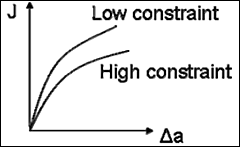Fig.1. Constraint effect on R-curves

The present paper focuses on the effect of constraint on the R-curve using constraint parameters (T-stress, Q-stress, A2). The J-A2 approach was developed by Yang et al and has been recently used to quantify the constraint effect on fracture toughness for different geometry and loading modes. In this paper, three constraint theories are first briefly reviewed. A framework to construct the R-curve is then developed and demonstrated. The predicted constraint-dependent R-curves are then compared against test results.

## 2. Review of constraint theories

### 2.1 J-T approach

The T-stress represents a stress parallel to the crack surface near the crack front. Although derived from linear elastic analysis of structures containing a crack under small-scale yielding (SSY), it has been shown that the T-stress can also be used in elastic-plastic fracture analysis. In terms of calculation, the T-stress approach requires only a linear-elastic analysis rather than an elastic-plastic analysis of the cracked structure. There is a considerable debate on the use of T-stress when large-scale yielding (LSY) occurs. Theoretically, the principle of T-stress is lost under LSY. However, Parks has shown that the T-stress can still be a corrector of near tip stress tri-axiality under this condition.

### 2.2 J-Q approach

The limitation of the T-stress approach in large scale yielding can be overcome by using a J-Q approach. The parameter Q is a hydrostatic or tri-axiality term in the stress field at the crack tip. Q can be defined based on either an HRR field or an SSY field. The Q factor may be expressed as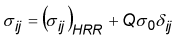or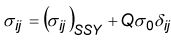where σ ij is the crack tip stress, σ0 is the yield stress and δ is the Kronecker delta. Numerical studies reveal that the use of the SSY field improves the radial independence of Q (therefore J-Q theory) compared to using a reference solution based on HRR. One deficiency of the Q approach is that Q is distance and load dependent under LSY. As a result, Q appears not to be a satisfactory constraint parameter in the analysis of ductile crack growth.

### 2.3 J-A2 approach

The J-A2 approach is based on the theoretical elastic-plastic asymptotic stress fields at the crack tip under plane strain conditions in a power-law hardening material. Yang et al demonstrated that the first three terms in the asymptotic series solution for the stress can characterize the crack tip mechanics fields within r/(J/σ0)=5 where r is the radial coordinate in the local polar coordinate system with the origin at the crack tip. These three terms however are not independent with each other and they can be expressed using two parameters. As an example, the stress near a crack tip using the J-A2 approach may be written as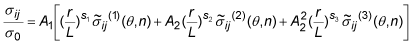where A1 and A2 are the two parameters used to characterise the crack tip stress field. The first term in the above expression corresponds to the stress from HRR field, ie,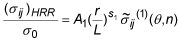where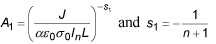in which α, n are the hardening parameters and ε0 is initial yield strain. The remaining two terms in Eq.3 refer to the higher order stress terms. The parameter In , stress power exponents sk and angular function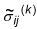(i, j, k=1,2,3) are only dependent of the hardening exponent and have been tabulated by Chao and Zhang. The parameter L is a characteristic length which can be chosen as crack length, specimen width, thickness or unity.

The value of A2 can be determined directly by comparing the calculated stress from finite element analysis (FEA) at the crack tip with Eq.3. Alternatively, A2 can be determined through the relationship between A2 and Q-stress which can be obtained by comparing the theoretical crack tip stresses predicted by J-Q theory with the stresses from J-A2 theory.

### 2.4 Bending specimens under large-scale yielding

The two-parameter characterisation of the elastic-plastic fields near the crack tip is suitable for shallow cracked specimens. However, these approaches break down in the case of deep bend specimens under LSY or fully plastic deformation because the global bending stress significantly alters the stress fields near the crack tip. A possible way to solve this problem is to develop a new parameter that characterizes the global bending stress near the crack tip. Methodologies in this category are referred to as three-parameter fracture mechanics in the present paper. These include J-Q-k2, J-Qt-Qp  and modified J-A2 theory.

In the J-Q-k2 theory, Q and k2 can be inferred from FEA results. However, it is not clear whether the parameter k2 is a constraint parameter or a loading parameter. The J-Qt-Qp theory is derived from J-Q theory where Q is decomposed into Qt (a distance independent term related to T-stress) and Qp (a distance dependent term related to the global bending stress field).

The modified J-A2 theory (J-A2-M) introduces an additional bending term M. According to Chao et al, the global bending stress is a linear function of the bending moment, and the opening stress in bending specimens is expressed as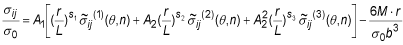where M is the moment per unit length, ie, the moment divided by the thickness of the specimen and b=W-a is the un-cracked ligament along the width direction where W is the width of the specimen and a is the crack depth.

## 3 Constraint Effect on R-curve

Without considering the constraint effect, the R-curve can be approximately fitted by a power-law relationship. The ASTM E-1820 gives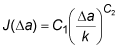where C1 and C2 are constants and k = 1.0 mm. Note that Eq.7 assumes J(Δa = 0) = 0. When the constraint effect is included, Eq.7 can be modified as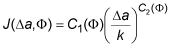where the constraint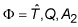depending on the constraint parameter to be chosen. The two functions C1 and C2 depend on the selected constraint parameter Φ. The non-dimensional constraint parameter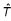Symbol.1.

is defined by normalising the T-stress by yield stress σ0, i.e.,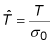The T-stress has been considered for R-curve modelling by Nyhus et al. Eq.8 provides a choice of T-stress, Q-stress and A2 for constrained based R-curve modelling. It should be noted that a similar mathematical expression which uses A2 as a constraint parameter was proposed by Chao and Zhu. The difference between the present development and the proposed method by Chao and Zhu is that Eq.8 provides a more general framework for constraint based R-curve modelling rather than focusing on a specific constraint parameter.

In order to mathematically determine the two functions C1 (Φ) and C2 (Φ) in Eq.8, the J-integral at two distinct crack extension lengths Δa1 and Δa2 must be known. Because Ja, Φ) is also a function of Φ, for a given crack extension length, the mathematical expression of J(Φ) can be accomplished by a curve fitting of the experimental data. It is recommended that a linear curve fitting shall be based on at least three data points. Therefore, at least three experimental R-curves at different constraint levels should be used to construct the theoretical R-curves. Fig.2 gives a schematic procedure to determine C1 (Φ) and C2 (Φ).

As shown in Fig.2a, assume that J-integral at the two crack extension lengths is given by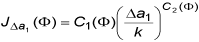[9a]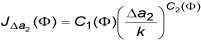[9b]

For each crack extension, there are three data points which can be used to fit a mathematical function JΔa (Φ) (Fig.2b). By rearranging Eq.9 and using the functions obtained from Fig. 2b we have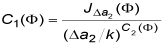[10a]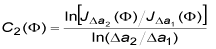[10b]

Specifically, when Δa2 = 1 mm, C1 (Φ) = J Δa2 (Φ). If JΔa1 (Φ) = a(Φ) and JΔa2 (Φ) = b(Φ), then . In other cases, the term In can be determined by using a set of Φ to obtain numerical result which can then be fitted as either a linear or a quadratic relation (Fig.2c). Once C1 (Φ) and C2 (Φ) are known for a given material, the R-curve can be predicted for cracked specimens with different constraints provided that the constraint Φ is known.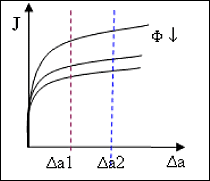Fig.2. R-curve modelling procedures

a)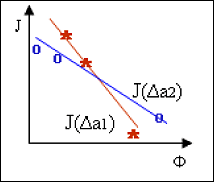b)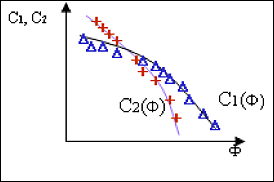c)

## 4 Application of constraint-based R-curve theories

### 4.1 R-curve testing

Test data measured by Joyce and Link were employed for demonstrating the above methodology. The test included a set of SENB and SENT specimens with a/W ratios varying from 0.1 to 0.7. The size of the specimens and the crack are given in Table 1 (constraint values are obtained from FEA, see Section 4.2). All specimens were side grooved after pre-cracking.

Table 1 Fracture toughness and constraint values

SpecimenTypea/WW, mmB, mmJIC, N/mmT/σ 0QA2
FYO 10SA SENT 0.35 64 25 187.6 -0.31 -0.51 -0.28
FYO 2SB SENT 0.40 64 25 148.0 -0.25 -0.43 -0.25
FYO 3SB SENT 0.47 64 25 217.0 -0.23 -0.42 -0.24
FYO 4SA SENT 0.65 64 25 227.5 0.22 -0.28 -0.19
FYO 26 SENB 0.13 50 25 163.7 -0.46 -0.49 -0.26
FYO 27 SENB 0.14 50 25 173.7 -0.43 -0.45 -0.25
FYO 150 SENB 0.61 50 25 145.1 0.29 0.14 0
FYO 151 SENB 0.61 50 25 129.7 0.29 0.13 0
FYO 21 SENB 0.14 50 50 177.2 -0.43 -0.45 -0.25

The test specimens were made of a high strength structural steel, HY-100. This material has a Young's modulus E =200GPa and a Poisson ratio ν 0.3. The tensile test showed that the 0.2% offset yield stress of HY-100 is σ0 =747MPa whereas the ultimate strength is 877MPa.

Joyce and Link gave a range of fracture resistance curves for HY-100 for the SENB and SENT specimens (Figures 3 and 4 respectively). These results show that deeply-cracked specimens (high constraint) tend to give a lower value of resistance compared with the shallow-cracked specimens (low constraint).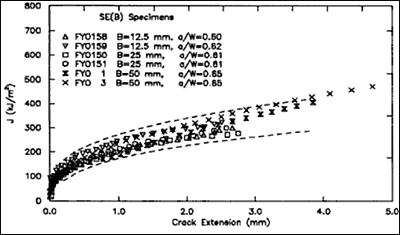Fig.3. R-curves for HY-100 for SENB specimens (Joyce and Link 1995)

Fig.3a) Deep-cracked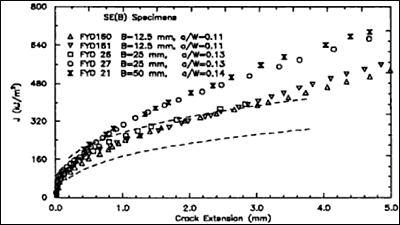Fig.3b) Shallow-cracked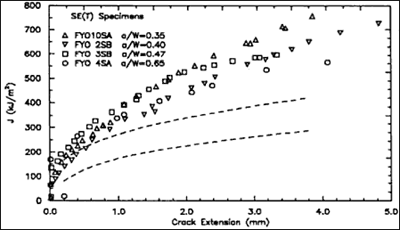Fig.4. R-curves for HY-100 for SENT specimens (Joyce and Link 1995)

### 4.2 Determination of constraint parameters using finite element analysis

Numerical simulation in the present work was carried out using ABAQUS 6.7 for the SENB and SENT cases described in section 4.1. Plane strain analyses were performed using eight-node quadratic elements with reduced integration (CPE8R). A focused mesh was used in the crack tip region. For the SENB specimens, a concentrated moment loading was applied on the two edges of the specimen. For the SENT specimens, a uniform distributed load was applied at the end.

The present analysis employed the Ramberg-Osgood power-law relation which is given by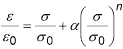where σ0 is the reference stress,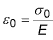is the reference strain, a is a material constant and n is the strain hardening coefficient. The strain hardening coefficient n 20 was determined based on an equation given in R6 . A J2 deformation theory of plasticity was used for multi-axial state deformation.

The T-stress was calculated by ABAQUS from an elastic analysis. A small scale yielding analysis was performed in order to determine the Q-stress and the parameter A2. The calculated constraint parameters T-stress, Q-stress and A2 are listed in Table 1. Note that the values of Q and A2 were taken at the distance 2σ0/J from the crack tip and the load was taken as that corresponding to crack initiation reported in .

### 4.3 Use of T-stress to construct the constraint corrected R-curve

Using the R-curves measured by Joyce and Link, the two functions C1Symbol.1. and C2Symbol.1. can be determined according to Eq.10. Take the two crack extension lengths Δa =0.2mm and Δa =1mm for example. The J-integral at Δa =0.2 mm and Δa =1mm as a function of T can be linearly fitted as[12a]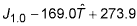[12b]

Substituting Eq.12 into Eq.10, we have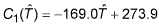[13a]

In Eq. 10b, the term In[J1.0(T)/J0.2(T)] can be numerically calculated with a set of . The function C2(T) is obtained by fitting the numerical results,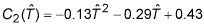[13b]

Substitute Eq.13 into Eq.8, we obtain the constraint-modified R-curve for HY-100,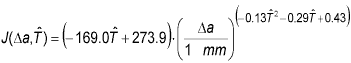Since the above R-curve contains the constraint parameter, Eq.14 can be used for an arbitrary cracked specimen once the constraint for that particular specimen is known. Fig.5 gives comparisons of the predicted R-curve using Eq.14 with the results measured for SENT and SENB specimens, respectively. It can be seen that the predictions from Eq.14 in general agree well with the measured results.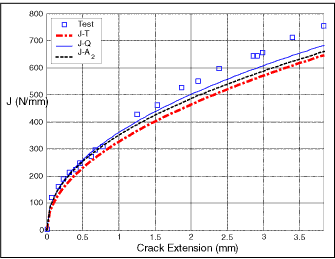Fig.5. Comparisons of predicted R-curves against the testing result

Fig.5a) a/W=0.35 (SENT)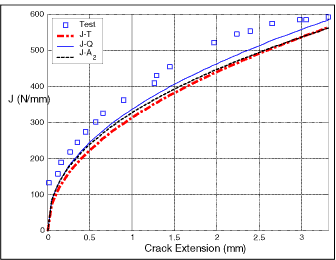Fig.5b) a/W=0.47 (SENT)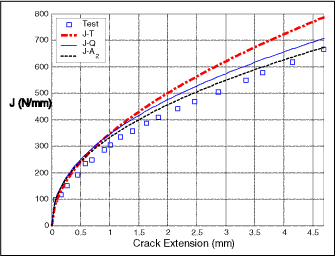Fig.5c) a/W=0.13 (SENB)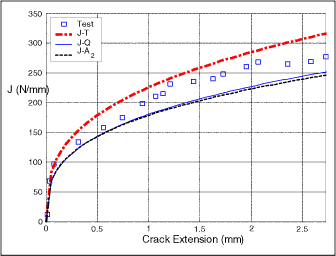Fig.5d) a/W=0.61 (SENB)

### 4.4 Use of Q to construct the constraint corrected R-curve

The procedure to construct the R-curve using the Q parameter is similar to that used for the T-stress. Again, take the two crack extension lengths Δa =0.2mm and Δa =1mm. The J-integral at Δa =0.2 mm and Δa =1mm as a function of Q can be linearly fitted as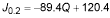[15a]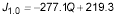[15b]

Using a similar procedure to that adopted to obtain Eq.13, the two functions C1(Q) and C2(Q) are given by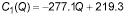[16a]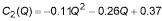[16b]

Substituting Eq.16 into Eq.8, the constraint-modified R-curve for HY-100 using Q stress is given by,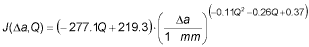The predicted R-curves using Eq.17 are also shown in Fig.5.

### 4.5 Use of A2 to construct the constraint corrected R-curve

In the case of A2, the J-integral at Δa =0.2 mm and Δa =1 mm as a function of A2 can be linearly fitted as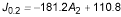[18a]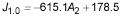[18b]

Following the same approach, the functions C1(A2) and C2(A2) can be given by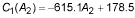[19a]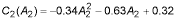[19b]

The R-curve using A2 is then given by,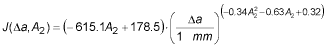## 5 Discussions

A comparison of the three predicted R-curves shows that all three parameters can be used to construct constraint-based R-curves. It has been argued that T-stress and Q-stress will fail under large scale yielding in bending-dominant specimens due to the presence of global bending stress which alters the crack tip stress field. Zhu and Leis developed a modified J-Q theory to construct constraint-dependent R-curves for this purpose. However, the present results show that R-curves predicted with T-stress and Q-stress are within up to about 20% of the test results (see Fig.5d for deeply-cracked SENB specimens). Further investigations are needed in order to clarify the validity of the J-Q theory in this region.

## 6 Conclusions

This paper has reviewed three constraint theories (T-stress, Q-stress and A2) for characterizing the fracture initiation toughness and fracture resistance curve. The three constraint parameters were used to construct constraint-corrected R-curves. An example was used to demonstrate the validity of the theory by comparing the predicted R-curves with the test data at different constraint levels. The method can be potentially used for engineering critical assessment of flaws where standard tests associated with high constraint give conservative R-curves.

## Acknowledgements

The authors wish to thank Professor Yuh J Chao, Department of Mechanical Engineering, University of South Carolina, USA for providing the report 'Tables of plane strain crack tip fields: HRR and higher order terms'.

## References

1. Pisarski HG and Wignall CM, Fracture toughness estimation for pipeline girth welds, Proceeding of IPC2002 International pipeline conference, (2002), Calgary, Canada
2. R6: Assessment of the Integrity of Structures Containing Defects, revision 4. 2001, British Energy Generation Ltd., UK
3. Joyce JA and Link RE, Effect of constraint on upper shelf fracture toughness Fracture Mechanics, ASTM STP-1256, 26, (1995) 142-177
4. Yang S, Chao YJ and Sutton MA, Higher order asymptotic crack tip fields in a power-law hardening material, Engineering Fracture Mechanics 45, (1993) 1-20
5. Chao YJ and Zhu XK, Constraint-modified J-R curves and its application to ductile crack growth, Journal of Fracture 106,(2000) 135-160
6. Parks DM, Advances in characterization of elastic-plastic crack-tip fields in topics in Fracture and Fatigue, Edited by AS Argon, (1992)59-98
7. Kirk MT, The second ASTM/ESIS symposium on constraint effects in fracture; an overview, International Journal of Pressure Vessel & Piping 64,(1995)259-275
8. Chao YJ and Zhang L, Tables of plane strain crack tip fields: HRR and higher order terms ME-Report, Department of Mechanical Engineering, University of South Carolina, (1997) 97-1
9. Wei Y and Wang T, Characterization of elastic-plastic fields near stationary crack-tip and fracture criterion, Engineering Fracture Mechanics 51, (1995) 541-553
10. Karstensen AD, Nekkal A and Hancock, JW, Constraint estimation for edge cracked bending bars, IUTAM Symposium on Nonlinear Analysis of Fracture, Kluwer Academic Publishers, Netherlands, (1997) 23-32
11. Chao YJ, Zhu XK and Kim Y, et al, Characterization of crack-tip field and constraint for bending specimens under large-scale yielding, International Journal of Fracture 127, (2004) 283-302
12. Nyhus, B, Zhang, ZL, Thaulow, C, Normalisation of material crack resistance curves by the T-stress, In: Proceedings of 14th European Conference on Fracture, 8-13 Sept, 2002, Cracow, Poland
13. Zhu XK and Leis BN, Bending modified J-Q theory and crack-tip constraint quantification, International Journal of Fracture 141, (2006) 115-134